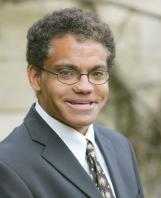# Invited Talks

## Titles and Abstracts

Mark van Hoeij
Florida State University, USA### Closed Form Solutions for Linear Differential and Difference Equations

Abstract:

Finding closed form solutions of differential equations has a long history in computer algebra. For example, the Risch algorithm (1969) decides if the equation y' = f can be solved in terms of elementary functions. These are functions that can be written in terms of exp and log, where "in terms of" allows for field operations, composition, and algebraic extensions. More generally, functions are in closed form if they are written in terms of commonly used functions. This includes not only exp and log, but other common functions as well, such as Bessel functions or the Gauss hypergeometric function. Given a differential equation L, to find solutions written in terms of such functions, one seeks a sequence of transformations that sends the Bessel equation, or the Gauss hypergeometric equation, to L.

Although random equations are unlikely to have closed form solutions, they are remarkably common in applications. For example, if y = \sum_{n=0}^{\infty} a_n x^n has a positive radius of convergence, integer coefficients a_n, and satisfies a second order homogeneous linear differential equation L with polynomial coefficients, then L is conjectured to be solvable in closed form. Such equations are common, not only in combinatorics, but in physics as well. The talk will describe recent progress in finding closed form solutions of differential and difference equations, as well as open questions.

Gabriele Nebe
RWTH Aachen, Germany### Computing with arithmetic groups

Abstract:

The classification of all finite simple groups is certainly the most important achievement in finite group theory of the last century. The knowledge of all building blocks of finite groups has let to the development of very powerful algorithms to constructively recognize finite matrix groups.

For infinite groups much less is known.

An important class of such groups are the $S$-arithmetic groups (such as GL_n(ZZ) or p_{2n}(ZZ[\frac{1}{2}])) also because of their connections to analytic and algebraic number theory. Fundamental finiteness properties for these groups have been proven by Borel and Serre by giving a locally finite CW complex on which the group acts faithfully. In joint work in progress with Renaud Coulangeon and Sebastian Schönnenbeck we replace the Borel-Serre space by a certain retract of a cone of positive forms to make it accessible for computations. This enables us to apply lattice theoretic algorithms to explicitely construct a finite set of matrices that generates the S-arithmetic group, as well as defining relations. Similar ideas are used in the community to study the integral homology of these groups.

James Worrell
University of Oxford, UK### Decision Problems for Linear Dynamical Systems

Abstract:

Linear dynamical systems, such as Markov chains, linear recurrence sequences, and linear differential equations, are both ubiquitous and extremely well-studied. Despite the apparent simplicity of the underlying dynamics, from the computational perspective there are many longstanding open problems. In this talk we focus in particular on problems relevant to automated verification, such as reachability, controllability, and termination. We describe recent progress in the area and survey some of the main open questions, including variants of the Skolem Problem for both discrete-time and continuous-time systems. We will also highlight relevant tools in number theory and real algebraic geometry that underly many of the positive results to date.